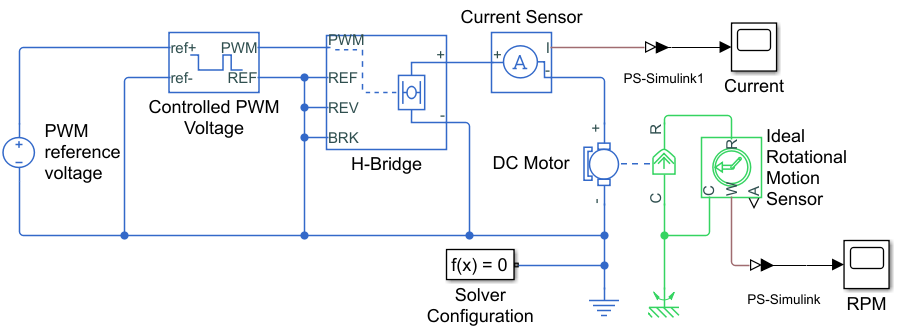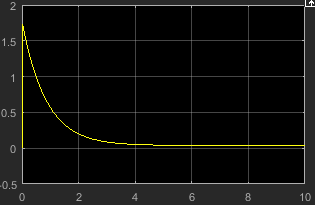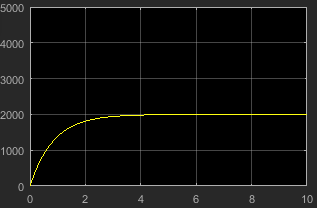## DC Motor Model

In this example, you model a DC motor driven by a constant input signal that approximates a pulse-width modulated signal and look at the current and rotational motion at the motor output.

To see the completed version of the model you create in this example, open the PWM-Controlled DC Motor example.

### Select Blocks to Represent System Components

Select the blocks to represent the input signal, the DC motor, and the motor output displays.

The following table describes the role of the blocks that represent the system components.

Block

Description

Solver Configuration

Defines solver settings that apply to all physical modeling blocks

Converts the input physical signal to a Simulink® signal

Controlled PWM Voltage

Generates the signal that approximates a pulse-width modulated motor input signal

H-Bridge

Drives the DC motor

DC Motor

Converts input electrical energy into mechanical motion

Current Sensor

Converts the electrical current that drives the motor into a measurable physical signal proportional to the current

DC Voltage Source

Generates a DC voltage

Electrical Reference

Provides the electrical ground

Mechanical Rotational Reference

Provides the mechanical ground

Ideal Rotational Motion Sensor

Converts the rotational motion of the motor into a measurable physical signal proportional to the motion

Scope

Displays motor current and rotational motion

### Build the Model

1. Create a new model.

2. Add the blocks in this table. The Library Path column specifies the hierarchical path to each block.

Block

Library

Quantity

Solver Configuration

Simscape > Utilities

1

Simscape > Utilities

2

Controlled PWM Voltage

Simscape > Electrical > Integrated Circuits

1

H-Bridge

Simscape > Electrical > Semiconductors & Converters > Converters

1

DC Motor

Simscape > Electrical > Electromechanical > Brushed Motors

1

Current Sensor

Simscape > Foundation Library > Electrical > Electrical Sensors

1

DC Voltage Source

Simscape > Foundation Library > Electrical > Electrical Sources

1

Electrical Reference

Simscape > Foundation Library > Electrical > Electrical Elements

1

Mechanical Rotational Reference

Simscape > Foundation Library > Mechanical > Rotational Elements

1

Ideal Rotational Motion Sensor

Simscape > Foundation Library > Mechanical > Mechanical Sensors

1

Scope

2

Note

You can use the Simscape™ function `ssc_new` with domain type `electrical` to create a Simscape model that contains these blocks:

• Scope

• Solver Configuration

• Electrical Reference

3. Rename and connect the blocks as shown in the diagram.Now you are ready to specify block parameters.

### Specify Model Parameters

Specify these parameters to represent the behavior of the system components:

#### Model Setup Parameters

These blocks specify model information that is not specific to a particular block:

• Solver Configuration

• Electrical Reference

• Mechanical Rotational Reference

As with Simscape models, you must include a Solver Configuration block in each topologically distinct physical network. This example has a single physical network, so use one Solver Configuration block with the default parameter values.

You must include an Electrical Reference block in each Simscape Electrical™ network. You must include a Mechanical Rotational Reference block in each network that includes electromechanical blocks. These blocks do not have any parameters.

#### Motor Input Signal Parameters

You generate the motor input signal using these blocks:

• The DC Voltage Source block (PWM reference voltage) generates a constant signal.

• The Controlled PWM Voltage block generates a pulse-width modulated signal.

• The H-Bridge block drives the motor.

In this example, all input ports of the H-Bridge block except the PWM port are connected to ground. As a result, the H-Bridge block behaves as follows:

• When the motor is on, the H-Bridge block connects the motor terminals to the power supply.

• When the motor is off, the H-Bridge block acts as a freewheeling diode to maintain the motor current.

In this example, you simulate the motor with a constant current whose value is the average value of the PWM signal. By using this type of signal, you set up a fast simulation that estimates the motor behavior.

1. In the DC Voltage Source block, set the Constant voltage parameter to `2.5` `V`.

2. Set the parameters of the Controlled PWM Voltage block:

• PWM frequency`4000` `Hz`

• Simulation mode`Averaged`

This value tells the block to generate an output signal whose value is the average value of the PWM signal. Simulating the motor with an averaged signal estimates the motor behavior in the presence of a PWM signal. To validate this approximation, use value of `PWM` for this parameter.

3. In the H-Bridge block, set the Simulation mode parameter to `Averaged`.

This value tells the block to generate an output signal whose value is the average value of the PWM signal. Simulating the motor with an averaged signal estimates the motor behavior in the presence of a PWM signal. To validate this approximation, use value of `PWM` for this parameter.

Note

The simulation mode for both the Controlled PWM Voltage and H-Bridge blocks must be the same.

#### Motor Parameters

Configure the block that models the motor.

Set the parameters of the DC Motor block, leaving the unit settings at their default values where applicable:

• Electrical Torque section:

• Model parameterization```By rated load and speed```

• Armature inductance`0.01` `H`

• No-load speed`4000` `rpm`

• Rated speed (at rated load)`2500` `rpm`

• Rated load (mechanical power)`10` `W`

• Rated DC supply voltage`12` `V`

• Mechanical section:

• Rotor inertia`0.0002` `kg*m^2`

• Rotor damping`0.5e-5` `N*m/(rad/s)`

#### Current Display Parameters

Specify the parameters of the blocks that create the motor current display:

• Current Sensor block

• Current scope

Of the three blocks, only the PS-Simulink Converter1 block has parameters. Set the PS-Simulink Converter1 block Output signal unit parameter to `A` to indicate that the block input signal has units of amperes.

#### Torque Display Parameters

Specify the parameters of the blocks that create the motor torque display:

• Ideal Rotational Motion Sensor block

• RPM scope

Of the three blocks, only the PS-Simulink Converter block has parameters you need to configure for this example. Set the PS-Simulink Converter block Output signal unit parameter to `rpm` to indicate that the block input signal has units of revolutions per minute.

Note

You must type this parameter value. It is not available in the drop-down list.

### Configure the Solver Parameters

Configure the solver parameters to use a continuous-time solver. Simscape Electrical models only run with a continuous-time solver when you clear the Local Solver parameter of a Solver Configuration block. Use the Configuration Parameters dialog box to change the simulation end time, tighten the relative tolerance for a more accurate simulation, and remove the limit on the number of simulation data points Simulink saves.

1. In the model window, select Modeling > Model Settings to open the Configuration Parameters dialog box.

2. In the left pane, click Solver:

• Set Stop time to `10`.

• Set Solver to ```ode15s (Stiff/NDF)```.

• Set Max step size to `1`.

• Set Relative tolerance to `1e-3`.

3. Click OK.

### Run the Simulation and Analyze the Results

Run the simulation and plot the results. In the model window, select Simulation > Run.

To view the motor current and torque, double-click the Scope blocks. You can do this before or after you run the simulation.

Note

By default, the scope displays appear stacked on top of each other on the screen, so you can only see one of them. Click and drag the windows to reposition them.

This figure shows the motor current.This figure shows the motor rpm.As expected, the motor runs at about 2000 rpm when the applied DC voltage is 2.5 V.